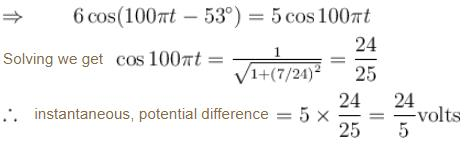Courses

# Alternating Current NAT Level - 2

## 10 Questions MCQ Test Topic wise Tests for IIT JAM Physics | Alternating Current NAT Level - 2

Description
This mock test of Alternating Current NAT Level - 2 for Physics helps you for every Physics entrance exam. This contains 10 Multiple Choice Questions for Physics Alternating Current NAT Level - 2 (mcq) to study with solutions a complete question bank. The solved questions answers in this Alternating Current NAT Level - 2 quiz give you a good mix of easy questions and tough questions. Physics students definitely take this Alternating Current NAT Level - 2 exercise for a better result in the exam. You can find other Alternating Current NAT Level - 2 extra questions, long questions & short questions for Physics on EduRev as well by searching above.
*Answer can only contain numeric values
QUESTION: 1

### In an AC circuit the potential differences across an inductance and resistance joined in series are respectively 16V and 20V. The total potential difference across the circuit is X/5. Then X is :

Solution: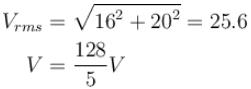*Answer can only contain numeric values
QUESTION: 2

### A LCR series circuit with 100Ω resistance is connected to an AC source of 200V and angular frequency 300 radians per second. When only the capacitance is removed, the current lags behind the voltage by 60°. When only the inductance is removed, the current leads the voltage by 60°. Calculate the average power dissipated in LCR circuit in watts.

Solution: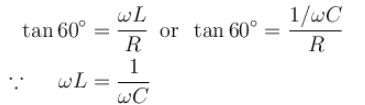Impedance of circuit = R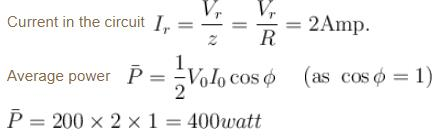*Answer can only contain numeric values
QUESTION: 3

### An inductor (XL = 2Ω) a capacitor (XC = 8Ω) and a resistance 8Ω are connected in series with an AC source. The voltage output of AC source is given by V = cos100πt,  then the impedance of the circuit (in Ω).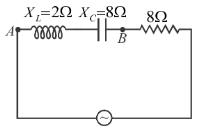Solution: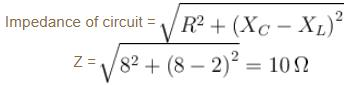*Answer can only contain numeric values
QUESTION: 4

LCR series, AC circuit operates at half its resonant frequency and at a power factor of 0.8. Then  the ratio of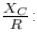Solution: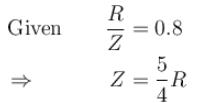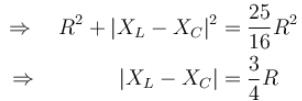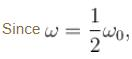resonant frequency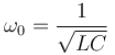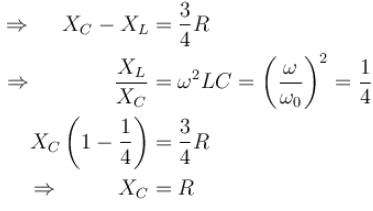*Answer can only contain numeric values
QUESTION: 5

In the LCR circuit shown if only L is removed, the current leads the supply voltage by 30°. If only C is removed the current lags the voltage by 60°. The resonant frequency is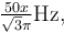then what is the value of x.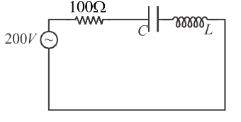Solution: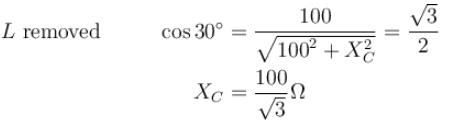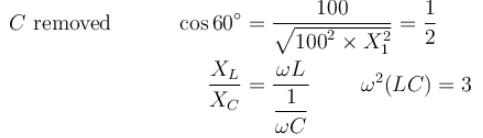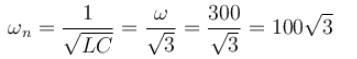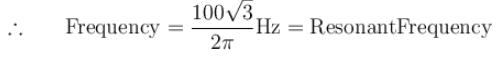*Answer can only contain numeric values
QUESTION: 6

The voltage time (V-t) graph for triangular wave having peak value V0 is as shown in figure. The rms value of V in time interval from t = 0  to T/4 is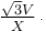Then, X is :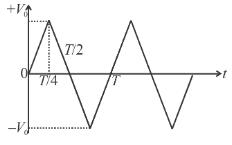Solution: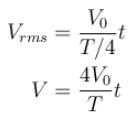⇒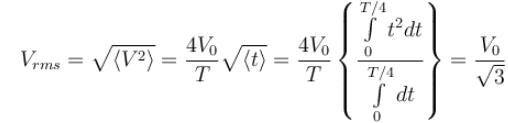*Answer can only contain numeric values
QUESTION: 7

The average value of current i = Im sin ωt from t = π/2ω to t = 3π/2ω is how many times of Im ?

Solution:*Answer can only contain numeric values
QUESTION: 8

In the circuit diagram shown, X= 100Ω, X= 200Ω and R = 100Ω. The effective current through the source is √N.  Then N is :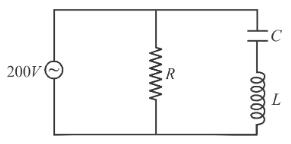Solution: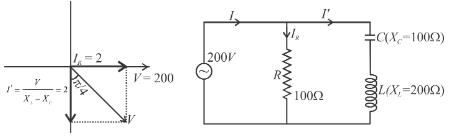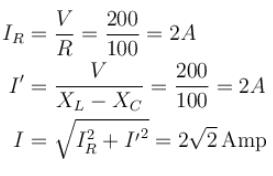*Answer can only contain numeric values
QUESTION: 9

Find the average value of current from t = 0 to t = 2π/ω, if the current varies as i = Imsinωt.

Solution: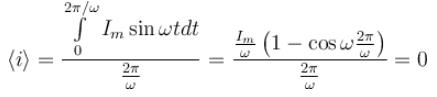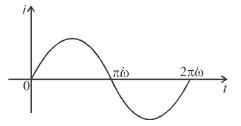It can be seen graphically that the area of i = t graph of one cycle is zero.
∴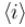is one cycle.

*Answer can only contain numeric values
QUESTION: 10

An inductor (XL = 2Ω), a capacitor (XC = 8Ω) and a resistance 8Ω are connected in series with an AC source. The instantaneous potential difference between A and B when it is half of the voltage output from source at that instant is x/5 volt, then x is.

Solution:

The current leads in phase by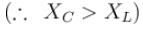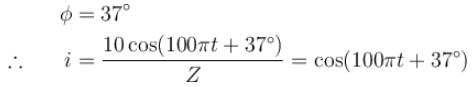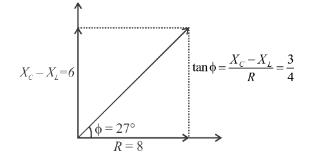The instantaneous potential difference across AB is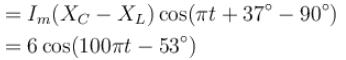The instantaneous potential difference across AB is half of source voltage.